ISSAC 97 - Meijer G Function Representations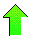Contents News View

 Properties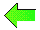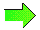The Meijer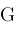function has various properties , , .  Among those of interest to us are:

Theorem.  (Basic Properties.)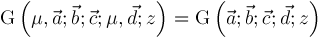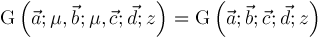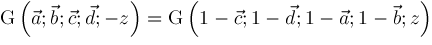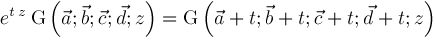Theorem.  (Duplication Formula.)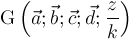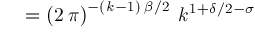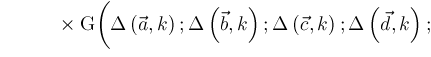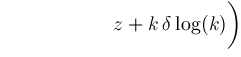where we use notation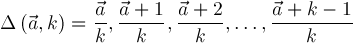Theorem.  (Slater's Theorem.)If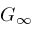converges and the elements of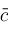are distinct mod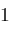, then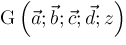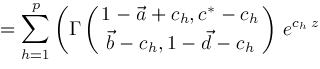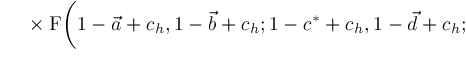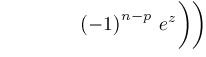where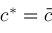with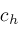omitted.©2004-2021 Planet Quantum Kelly Roach